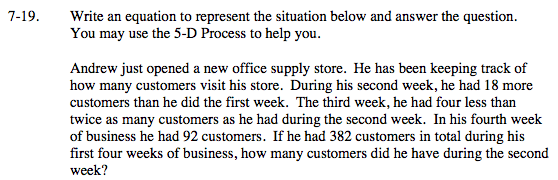### Home > MC2 > Chapter 7 > Lesson 7.1.2 > Problem7-19

7-19.Create an expression to represent this problem using a variable, x. Try to write and solve for an equation, or if necessary, use the 5-D Process you learned earlier.

Each of the weeks ultimately relates to the first week, so we can define the first week's customers as x. Write expressions for each of the other weeks based on the variable.

First week : x
Second week: x + 18
Third Week: 2(x + 18) − 4
Fourth Week: 92

Create an expression by summing up each of the week's customers to total 382 as given by the problem, then solve for the variable. Remember, the second week's customers was 18 more than the first week's (x).

78 customers